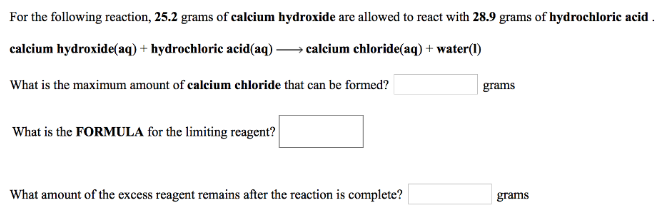# Problem: For the following reaction, 25.2 grams of calcium hydroxide are allowed to react with 28.9 grams of hydrochloric acid. calcium hydroxide (aq) + hydrochloric acid (aq) → calcium chloride (aq) + water (l) What is the maximum amount of calcium chloride that can be formed? What is the FORMULA for the limiting reagent? What amount of the excess reagent remains after the reaction is complete?

###### FREE Expert Solution
82% (413 ratings)###### Problem Details

For the following reaction, 25.2 grams of calcium hydroxide are allowed to react with 28.9 grams of hydrochloric acid.

calcium hydroxide (aq) + hydrochloric acid (aq) → calcium chloride (aq) + water (l)

What is the maximum amount of calcium chloride that can be formed?

What is the FORMULA for the limiting reagent?

What amount of the excess reagent remains after the reaction is complete?